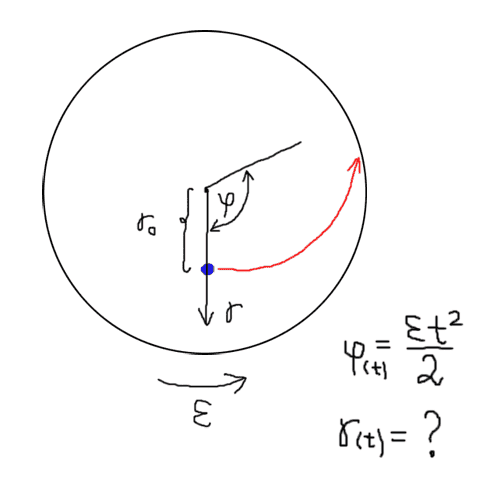# Curved motion, r(φ) when r is accelerating

Hi there!

I have a problem to find an equation of r(t), which will help me to describe motion of blue dot. I have seen many cases where there is a linear motion and you can write φ=ωt , r=V0t, but here there is an acceleration. I think I can write φ=εt2/2, but what about r(t) ? I have tried differencial equation
r = at2/2 + r0 → r = (r''-rφ')t2/2 + r0, but not sure if it's right. I need all this to have equation of path r(φ). r0 is initial position of blue dot, where V0=0 and ω=0. Cheers!Last edited:

Stephen Tashi
Hi there!

I have a problem to find an equation of r(t), which will help me to describe motion of blue dot.

There are many possible formulas for r(t) that would produce a curved trajectory. To pose a specific mathematical problem, you have to state a complete set of requirements for r(t). Is this a physics problem? Are forces involved?

There are many possible formulas for r(t) that would produce a curved trajectory. To pose a specific mathematical problem, you have to state a complete set of requirements for r(t). Is this a physics problem? Are forces involved?

I am trying to describe a motion of a clay target in a spring-powered target thrower. You can involve some forces (f.ex. friction between target and friction tunnel of an arm), but this complicates case even more. The only constant I can assume is velocity when target leaves the arm (27m/s). Everything else has to be calculated. The main thing is to have ε of this arm, because then from M=I⋅ε we can have required force in a spring.

Stephen Tashi
The instantaneous velocity in polar coordinates is given by the vector $\frac{dr}{dt}r$ in the radial direction and $\frac{d\theta}{dt} \theta$ in the tangential direction. If you want the magnitude of this velocity vector to be 27 m/sec at the time t when the target reaches the end of the arm, you have to keep in mind the contribution of the component $\frac{dr}{dt} r$ to the velocity. It will be important to have a physically reasonable formula for $r(t)$.

mfb
Mentor
You can involve some forces (f.ex. friction between target and friction tunnel of an arm), but this complicates case even more.
Without forces, how would you know how the object moves?
Everything else has to be calculated.
There are many possible motions that end with a speed of 27m/s.

A.T.